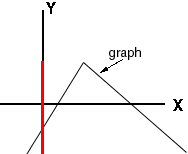Sender: Tara Doucet Hi my name is Tara, I have two math problems that I need help with in my calculus math class. If f(x)= x - 2 show that (x+3)f(x)-(x+2)f(x+1)+4=0 Graph this function and use the graph to determine the range y=2x2 - 8x - 3 I hope you can help, I would be very grateful. I am a student, at Nipissing university. Hi Tara, The way we write functions describes how they work. f(x) = x - 2 describes the function that "subtracts 2". By that I mean that whatever number you give to the function, it subtracts 2. Thus if you give it 5 then it returns 5 - 2 = 3, ie f(5) = 5 - 2 = 3. Likewise f(-8) = -8 - 2 = -10, f(5 + 1/2) = 5 + 1/2 - 2 = 3 1/2 and f(x + 1) = x + 1 - 2 = x - 1. Substitute f(x) = x - 2 and f(x + 1) = x - 1 into (x+3)f(x)-(x+2)f(x+1)+4, expand and simplify to see if you get zero. If you have the graph of a function then the range is the set of all Y-corrdinates of points on the graph. If the graph is given in the diagram below then the range is the part of the Y-axis that is coloured red.Cheers, Harley

Go to Math Central